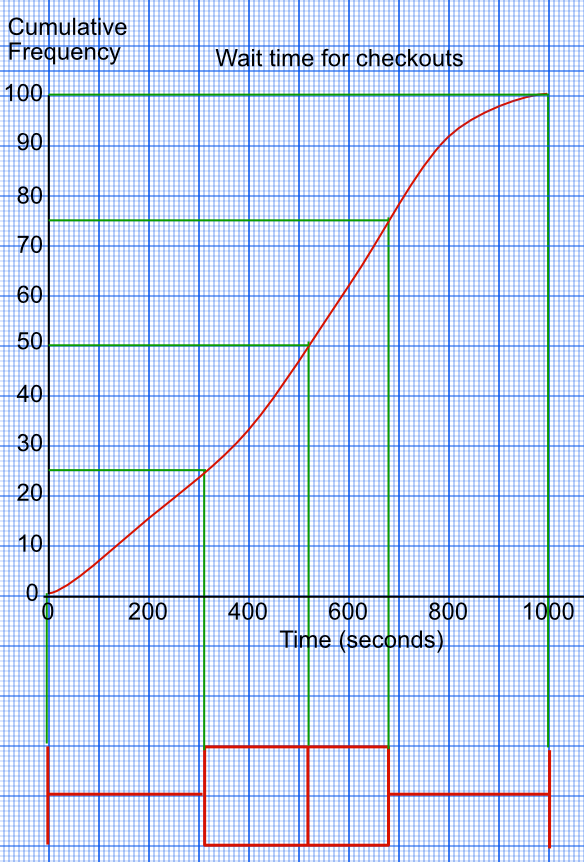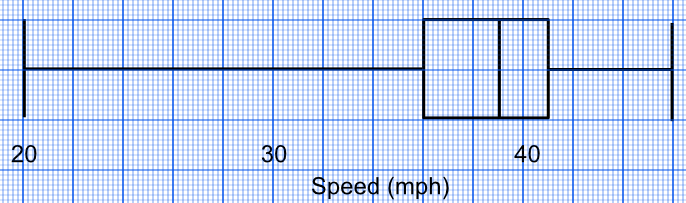Box plots

# Box plots

GCSE(F), GCSE(H),

A Box Plot gives a visual presentation of the median and spread of a set of data. The Box Plot shows the Range, Inter-Quartile Range and the Mean.

It is also known as a Box and Whisker Diagram.

The diagram consists of 5 pieces of information. The Lowest Value is taken from the lowest value in the data set; similarly the Highest Value. The Median is the middle value when placed in order; the Lower Quartile is the frac((n + 1))(4)th value (reading from the lowest value) and the Upper Quartile is the frac(3(n + 1))(4)th value (also when reading from the lowest value).A Box plot can also be drawn from a Cumulative Frequency diagram.Box Plots give more information on the spread of data, which is useful when comparing sets of data:
• a compact box, with long whiskers, shows the probable presence of outliers;
• a compact box, with short whiskers, shows a consistent set of data;
• an extended box, with long whiskers, shows a wide range of inconsistent data;
• an extended box, with short whiskers, shows a consistent set of data over a wide range.

## Examples

1. Draw a Box plot for the following information, which is the speed of 27 cars through a radar trap in a 40mph zone:
34, 36, 37, 37, 33, 42, 44, 35, 46, 33, 38, 39, 40, 41, 20, 44, 38, 37, 42, 36, 41, 40, 40, 39, 40, 39, 39

Answer:Sort the data into order: a Stem-and-Leaf diagram could be used: 2 | 0
3 | 3 3 4 5 6 6 7 7 7 8 8 9 9 9 9
4 | 0 0 0 0 1 1 2 2 4 4 6
Key 2|0 represents 20mph

Lowest Value is 20

Highest Value is 46

Median Value is the 13th value, which is 39

Lower Quartile is frac((27+1))(4) = 7th car = 36

Upper Quartile is 3frac(27+1)(4) = 21st car = 41 giving a diagram:

2. Two classes were each given a test paper. The information was shown on a box plot. Make two comparisons between the results from class A and the results from class B.Answer: Class B had a higher median score.

Class B also had a smaller interquartile range.

Use a comparison of both the average and the range.